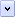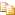JScript objects are collections of properties and methods. A method is a function that is a member of an object. A property is a value or set of values (in the form of an array or object) that is a member of an object. JScript supports four kinds of objects: intrinsic objects, objects you create, host objects, which are provided by the host (such as window and document in Internet Explorer) and Active X objects (external components).

# Objects as Arrays

In JScript, objects and arrays are handled almost identically. Both can have arbitrary properties assigned to them, and indeed Arrays are merely a special kind of Object. The difference between Arrays and Objects is that arrays have a "magic" length property, whilst objects do not. This means that if you assign a value to an element of an array that is greater than every other element — for example, myArray = "hello" — then the length property will automatically be updated to be 101 (the new length). Similarly, if you modify the length property of an array, it will delete any elements that are no longer part of the array.

All objects in JScript support "expando" properties, or properties that can be added and removed dynamically at run time. These properties can have any name, including numbers. If the name of the property is a simple identifier<<ref for identifier rules>>, it can be written after the object name with a period, such as:Copy Code
```var myObj = new Object();

// Add two expando properties, 'name' and 'age'
myObj.name = "Fred";
myObj.age = 42;```

If the name of the property is not a simple identifier, or it is not known at the time you write the script, you can use an arbitrary expression inside square brackets to index the property. The names of all expando properties in JScript are converted to strings before being added to the object.Copy Code
```var myObj = new Object();

// Add two expando properties that cannot be written in the
// object.property syntax.
// The first contains invalid characters (spaces), so must be
// written inside square brackets.
myObj["not a valid identifier"] = "This is the property value";

// The second expando name is a number, so it also must
// be placed inside square brackets
myObj = "100";```

Traditionally, array elements are given numeric indices, starting at zero. It is these elements that interact with the length property. Nevertheless, because all arrays are also objects, they support expando properties as well. Note, though, that expando properties do not interact with the length property in any way. For example:Copy Code
```// An array with three elements
var myArray = new Array(3);

myArray = "Hello";
myArray = 42;
myArray = new Date(2000, 1, 1);

// This will display 3, the length of the array

myArray.expando = "JScript!";
myArray["another Expando"] = "Windows";

// This will still display 3, since the two expando properties
// don't affect the length.

Although JScript does not directly support multi-dimensional arrays, you can store any sort of data inside array elements — including other arrays. So you can get the behavior of multi-dimensional arrays by storing arrays within the elements of another array. For example, the following code builds a multiplication table for the numbers up to 5:Copy Code
```// Change this number for a bigger table
var iMaxNum = 5;
// Loop counters
var i, j;

// New array. Make it iMaxNum + 1 because arrays start
// counting at zero, not 1.
var MultiplicationTable = new Array(iMaxNum + 1);

// Loop for each major number (each row in the table)
for (i = 1; i <= iMaxNum; i++)
{
// Create the columns in the table
MultiplicationTable[i] = new Array(iMaxNum + 1);

// Fill the row with the results of the multiplication
for (j = 1; j <= iMaxNum; j++)
{
MultiplicationTable[i][j] = i * j;
}
}